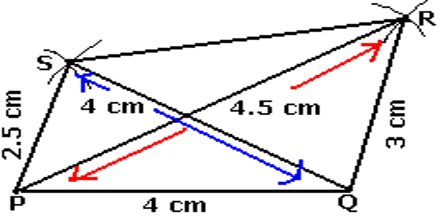Mathematic

# lecture on Constructing a QuadrilateralGeneral purpose of this lecture is to present on Constructing a Quadrilateral. Suppose, Construct quadrilateral ABCD, given that = 7cm, = 4cm and. Step :- (1) Draw a the line segment = 7cm. (2) With A as centre and radius 4 cm cut an arc on the line. (3) At B draw an angle of . (4) At D draw an angle of with a protractor. Construction:- When four sides and one diagonal are given; when two diagonals and three sides are given; when two adjacent sides and three angles are given; when three sides and two included angles are given and when other special properties are known.